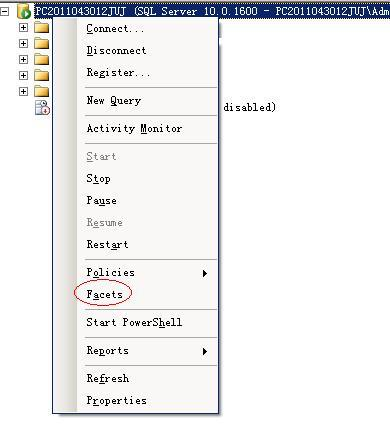# SQL存储过程解密研究

1、并没有删除原存储重建，仅仅在控制台做了一个输出，拷贝出来很不方便。
2、对于长度大点的加密数据会解密失败。sqlcmd -A -S 192.168.1.101 -U sa -P 123456

>USE TEST
>GO

select @maxColID = max(subobjid),@intEncrypted = imageval FROM sys.sysobjvalues WHERE objid = object_id(@procedure)

DECLARE @real_01 nvarchar(max)
DECLARE @fake_01 nvarchar(max)
DECLARE @fake_encrypt_01 nvarchar(max)
DECLARE @real_decrypt_01 nvarchar(max)

WHILE @intProcSpace<=(datalength(@real_01)/2)
BEGIN
--xor real & fake & fake encrypted
SET @real_decrypt_01 = stuff(@real_decrypt_01, @intProcSpace, 1, NCHAR(UNICODE(substring(@real_01, @intProcSpace, 1)) ^ (UNICODE(substring(@fake_01, @intProcSpace, 1)) ^ UNICODE(substring(@fake_encrypt_01, @intProcSpace, 1)))))
SET @intProcSpace=@intProcSpace+1
END

DECLARE @real_decrypt_01 nvarchar(max)
SET @real_decrypt_01 = replicate(N'A', (datalength(@real_01) /2 ))

SET @real_decrypt_01 = replicate(CONVERT(NVARCHAR(MAX), N'A'), (datalength(@real_01) /2 ))

CREATE TABLE [dbo].[SQL_DECODE](    [ID] [int] IDENTITY(1,1) NOT NULL,    [SQLTEXT] [nvarchar](max) NOT NULL, CONSTRAINT [ID] PRIMARY KEY CLUSTERED (    [ID] ASC)) ON [PRIMARY]GO

CREATE PROCEDURE [dbo].[DECODE_DATABASE]ASSET NOCOUNT ONBEGIN    DECLARE @PROC_NAME VARCHAR(256)    SET @PROC_NAME = ''    DECLARE @ROWS INT    DECLARE @TEMP TABLE(        NAME VARCHAR(256)    )    INSERT INTO @TEMP SELECT NAME FROM sysobjects WHERE TYPE = 'P'     AND NAME NOT IN (        'DECODE_DATABASE', 'DECODE_PROC'    )    SET @ROWS = @@ROWCOUNT    WHILE @ROWS > 0    BEGIN        SELECT @PROC_NAME = NAME FROM (            SELECT ROW_NUMBER() OVER (ORDER by NAME) AS ROW, NAME FROM @TEMP         ) T         WHERE ROW = @ROWS        EXEC [DECODE_PROC] @PROC_NAME        PRINT @PROC_NAME        SET @ROWS = @ROWS - 1    END        RETURN        EXEC master..xp_cmdshell 'bcp "SELECT [SQLTEXT] FROM TEST.dbo.[SQL_DECODE]" queryout C:\decode.txt -c -T -S PC2011043012JUJ'ENDGO

CREATE PROCEDURE [dbo].[DECODE_PROC](    @PROC_NAME SYSNAME = NULL)ASSET NOCOUNT ONDECLARE @PROC_NAME_LEN INT    --存储过程名长度DECLARE @MAX_COL_ID SMALLINT    --最大列IDSELECT @MAX_COL_ID = MAX(subobjid) FROM sys.sysobjvalues WHERE objid = OBJECT_ID(@PROC_NAME) GROUP BY imagevalSELECT @PROC_NAME_LEN = DATALENGTH(@PROC_NAME) + 29DECLARE @REAL_01 NVARCHAR(MAX)    --真实加密存储过程数据DECLARE @FACK_01 NVARCHAR(MAX)    --修改为假的存储过程，长度（40003 - 存在过程名长度），原理不明？DECLARE @FACK_ENCRYPT_01 NVARCHAR(MAX)    --伪加密存储过街程数据DECLARE @REAL_DECRYPT_01 NVARCHAR(MAX)    --最终解密后的数据，初始化为原始加密长度的一半的“A”，原理不明？SET @REAL_01 = (    SELECT imageval FROM sys.sysobjvalues WHERE objid = object_id(@PROC_NAME) AND valclass = 1 AND subobjid = 1)DECLARE @REAL_DATA_LEN BIGINTSET @REAL_DATA_LEN = DATALENGTH(@REAL_01)--PRINT @REAL_DATA_LENDECLARE @FACK_LEN BIGINTSET @FACK_LEN = @REAL_DATA_LEN * 10    --改造：假的长度在原真实数据长度上放大10倍--此处需将NVARCHAR显示转换成NVARCHAR(MAX)，不然将只能产生4K长度SET @FACK_01 = 'ALTER PROCEDURE ' + @PROC_NAME + ' WITH ENCRYPTION AS ' + REPLICATE(CONVERT(NVARCHAR(MAX), '-'), @FACK_LEN - @PROC_NAME_LEN)--PRINT '@FACK_01 = ' + STR(LEN(@FACK_01))EXECUTE (@FACK_01)SET @FACK_ENCRYPT_01 = (    SELECT imageval FROM sys.sysobjvalues WHERE objid = object_id(@PROC_NAME) AND valclass = 1 AND subobjid = 1)SET @FACK_01 = 'CREATE PROCEDURE ' + @PROC_NAME + ' WITH ENCRYPTION AS ' + REPLICATE(CONVERT(VARCHAR(MAX), '-'), @FACK_LEN - @PROC_NAME_LEN)SET @REAL_DECRYPT_01 = REPLICATE(CONVERT(NVARCHAR(MAX), N'A'), (DATALENGTH(@REAL_01) /2))--PRINT 'LEN(@REAL_DECRYPT_01) = ' + STR(LEN(@REAL_DECRYPT_01))--按位对 @REAL_01、 @FACK_01、 @REAL_DECRYPT_01 进行异或操作。DECLARE @INT_PROC_SPACE BIGINTSET @INT_PROC_SPACE = 1WHILE @INT_PROC_SPACE <= (DATALENGTH(@REAL_01) /2 )BEGIN    SET @REAL_DECRYPT_01 = STUFF(        @REAL_DECRYPT_01,         @INT_PROC_SPACE,         1,         NCHAR(UNICODE(SUBSTRING(@REAL_01, @INT_PROC_SPACE, 1)) ^ (UNICODE(SUBSTRING(@FACK_01, @INT_PROC_SPACE, 1)) ^ UNICODE(SUBSTRING(@FACK_ENCRYPT_01, @INT_PROC_SPACE, 1))))    )    SET @INT_PROC_SPACE = @INT_PROC_SPACE + 1END--移除WITH ENCRYPTIONSET @REAL_DECRYPT_01 = REPLACE(@REAL_DECRYPT_01, 'WITH ENCRYPTION', '')INSERT INTO [SQL_DECODE] VALUES (@REAL_DECRYPT_01)--PRINT '@REAL_DECRYPT_01 = ' + @REAL_DECRYPT_01--PRINT 'LEN(@REAL_DECRYPT_01) = ' + STR(LEN(@REAL_DECRYPT_01))--删除原存储过程SET @FACK_01 = 'DROP PROCEDURE ' + @PROC_NAMEEXEC(@FACK_01)GO

>EXEC [DECODE_DATABASE]
>GO